CBSE Class 10 Sample Paper for 2022 Boards - Maths Standard [Term 2]

Class 10
Solutions of Sample Papers for Class 10 Boards

## Mrs. Garg recorded the marks obtained by her students in the following table. She calculated the modal marks of the students of the class as 45. While printing the data, a blank was left. Find the missing frequency in the table given below

This question is similar to Question 33 CBSE Class 10 Sample Paper 2021 Boards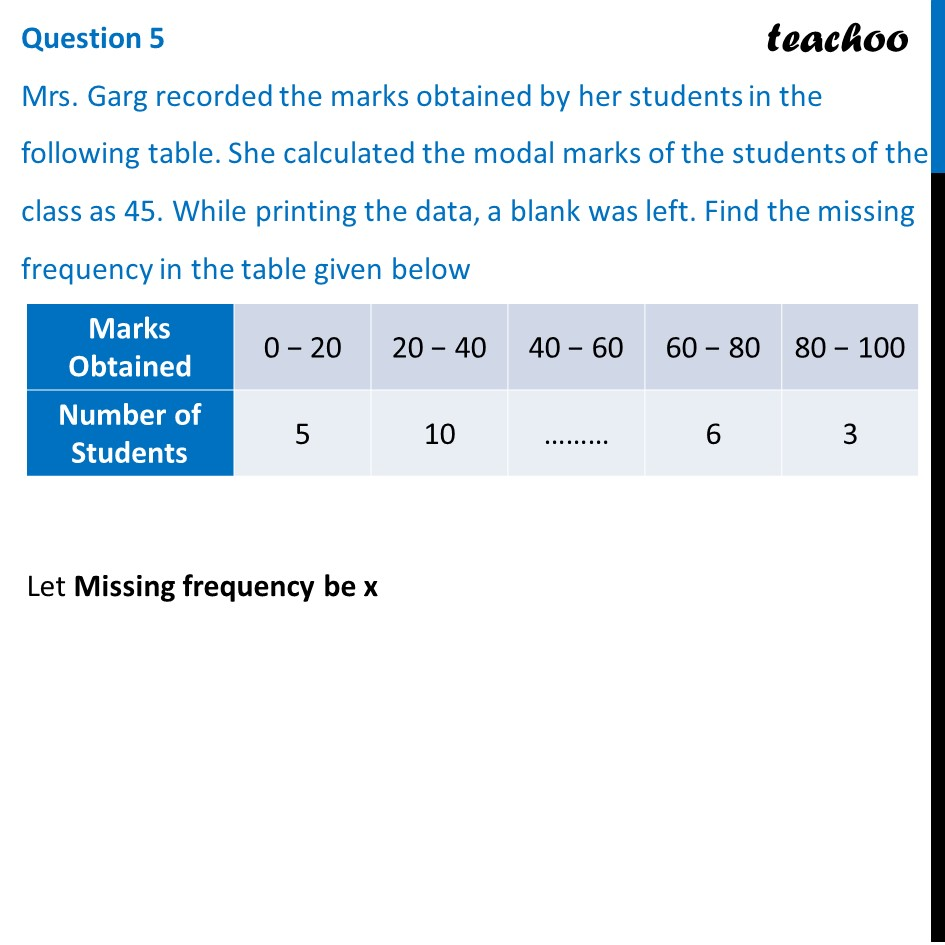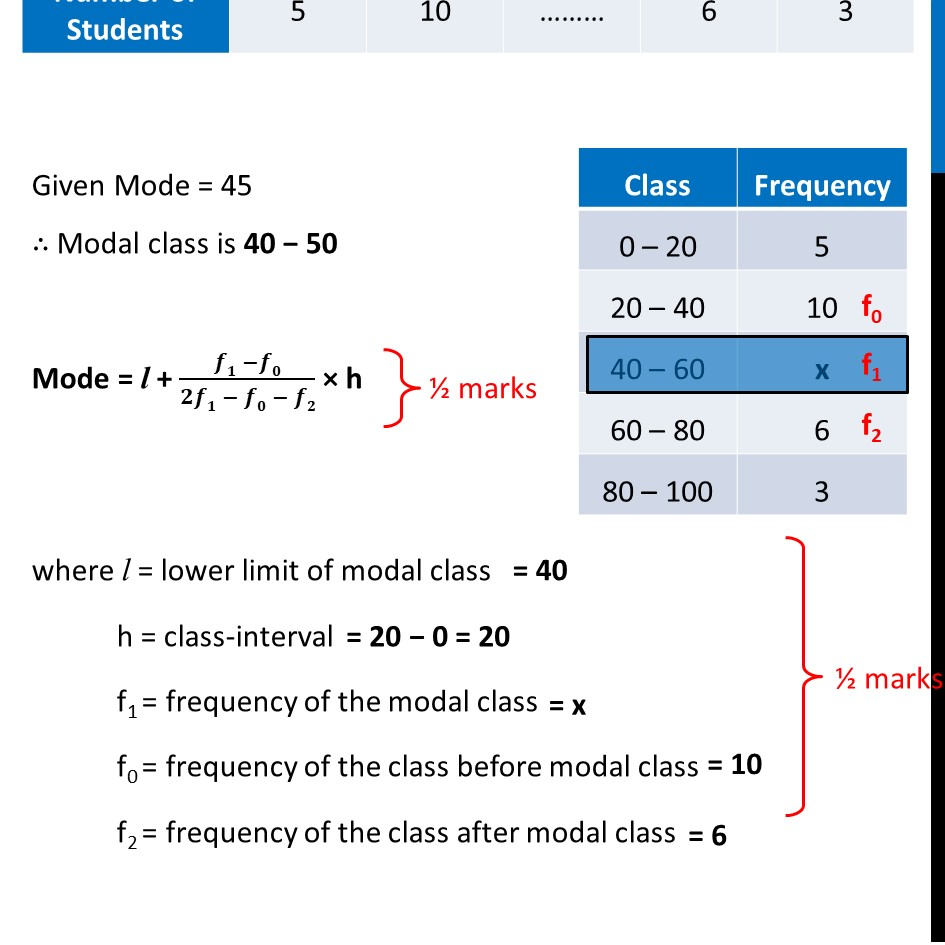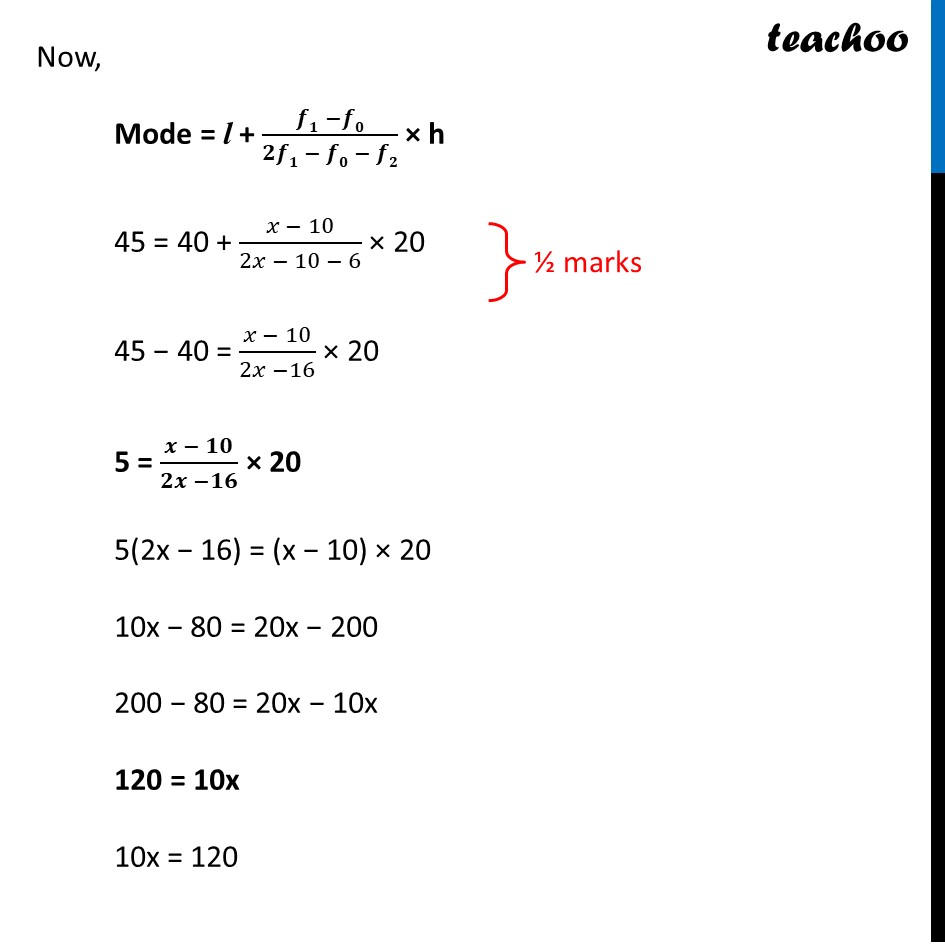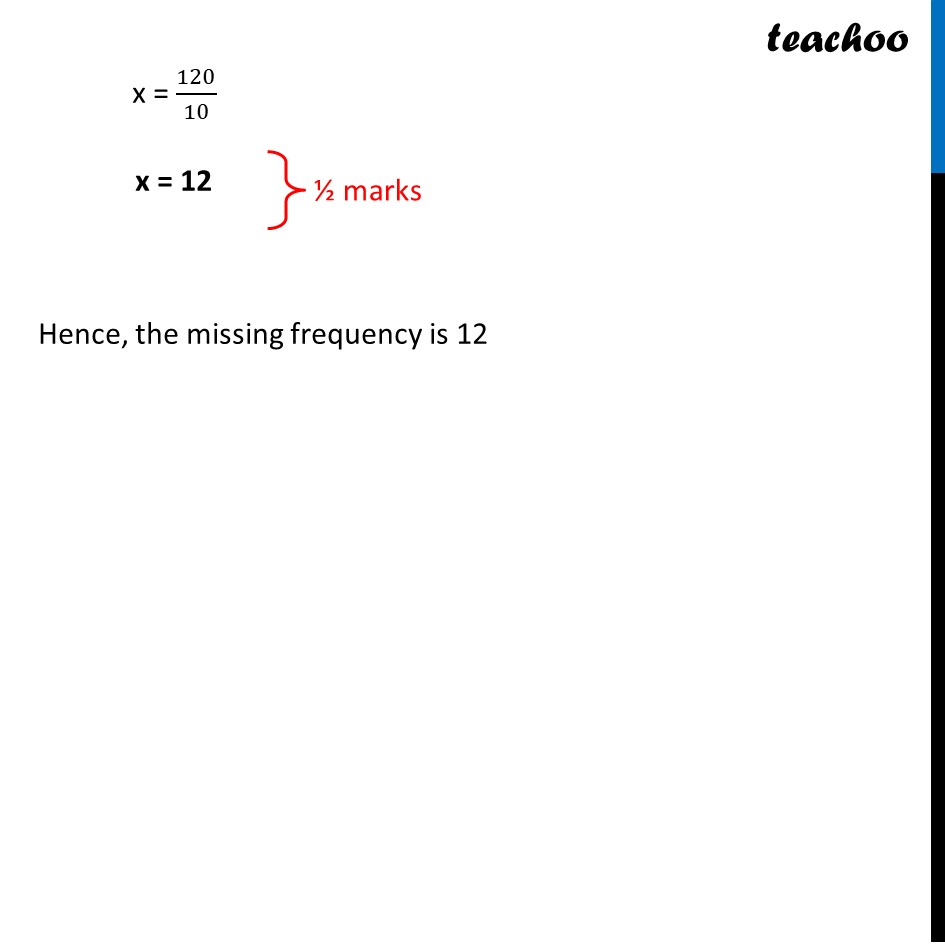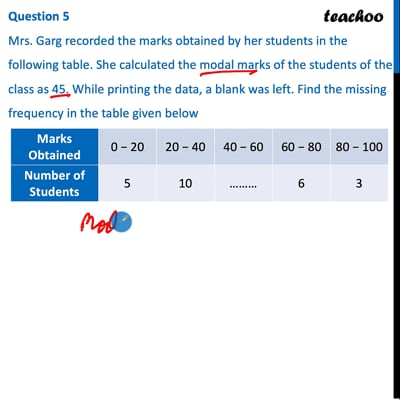This video is only available for Teachoo black users

### Transcript

Question 5 Mrs. Garg recorded the marks obtained by her students in the following table. She calculated the modal marks of the students of the class as 45. While printing the data, a blank was left. Find the missing frequency in the table given below Let Missing frequency be x Given Mode = 45 ∴ Modal class is 40 − 50 Mode = l + (𝒇𝟏 −𝒇𝟎)/(𝟐𝒇𝟏 − 𝒇𝟎 − 𝒇𝟐) × h where l = lower limit of modal class h = class-interval f1 = frequency of the modal class f0 = frequency of the class before modal class f2 = frequency of the class after modal class Now, Mode = l + (𝒇𝟏 −𝒇𝟎)/(𝟐𝒇𝟏 − 𝒇𝟎 − 𝒇𝟐) × h 45 = 40 + (𝑥 − 10)/(2𝑥 − 10 − 6) × 20 45 − 40 = (𝑥 − 10)/(2𝑥 −16) × 20 5 = (𝒙 − 𝟏𝟎)/(𝟐𝒙 −𝟏𝟔) × 20 5(2x − 16) = (x − 10) × 20 10x − 80 = 20x − 200 200 − 80 = 20x − 10x 120 = 10x 10x = 120 x = 120/10 x = 12 Hence, the missing frequency is 12